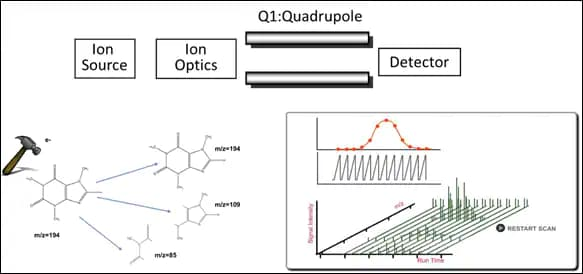# Environmental Solutions

## Shimadzu Gas Chromatography Mass Spectrometry (GCMS)

Gas Chromatography mass spectrometry is the workhorse of the organic environmental laboratory. This is the instrument you will use for methods such as 524.2, 624, 8260 volatiles, and 525, 625, and 8270 semi-volatiles. GCMS has a long history in the environmental laboratory and are often considered a commodity; however, there have been many advancements in the technology.

A GCMS consists of a GC with an injector, and column. The detector is a mass selective device, usually a quadrupole. There are subtle differences in the mass spectrometry detectors of each manufacturer. Rest assured, Shimadzu GCMS instruments are fully capable of running the EPA methods.

The mass spec detector ionizes the sample. In most environmental methods, the ionization is by electron impact at 70 electron volts. The ionization splits the analyte into distinctive, very reproducible mass fragments. These fragments accelerate through the quadrupole to an electron multiplier detector. The data output is a fragmentation pattern that can be library searched. Each compound has its own pattern of mass and relative abundance. Quantitation is by measuring intensity of mass. Qualitative ID is by retention time and how well the fragmentation pattern matches the pattern of a known standard or library.Before you can run environmental methods by GCMS, you must adjust the mass fragment pattern. This requirement is to guarantee that all laboratories obtain equivalent, reproducible spectra. You adjust the "tune" to meet method criteria for BFB (volatiles) or DFTPP (semi-volatiles) criteria. Confirm from the manufacturer that the instrument can meet the EPA tune criteria for the method you need to run.

The BFB criteria for EPA volatiles are similar with a few notable exceptions. Table 1 compares the BFB relative abundance criteria for six different EPA methods. Method 524.3/524.4 is the least stringent and methods 524.2, 624, and 8260c are the most stringent. If you always pass the most stringent criteria, you will also pass the least stringent.

Relative Abundance Criteria
Mass
(m/z)
Method 524.2 Method
524.3/M524.4
Method 624 Method 8260C CLP-SOW
50
15 to 40% of 95
NA
15 to 40% of 95
15 to 40% of 95
15 to 40% of 95
75
30 to 80% of 95
NA
30 to 60% of 95
30 to 60% of 95
30 to 80% of 95
95
Base Peak, 100%
Base Peak, 100%
Base Peak, 100%
Base Peak, 100%
Base Peak, 100%
96
5 to 9% of 95
5 to 9% of 95
5 to 9% of 95
5 to 9% of 95
5 to 9% of 95
173
<2% of 174
<2% of 174
<2% of 174
<2% of 174
<2% of 174
174
>50% of 95
>50% of 95
>50% of 95
>50% of 95
50 to 120% of 95
175
5 to 9% of 174
5 to 9% of 174
5 to 9% of 174
5 to 9% of 174
4 to 9% of 174
176
>95 to <101% of 174
>95 to <105% of 174
>95 to <101% of 174
>95 to <101% of 174
>95 to <101% of 174
177
5 to 9% of 176
5 to 10% of 176
5 to 9% of 176
5 to 9% of 176
5 to 9% of 176

Table 2 compares the DFTPP for five different EPA methods. Method 525.3 is the least stringent followed by Method 525.2. Methods 625 and 8270D are the most stringent. Similar to BFB, if you pass the criteria for the most stringent method, you will also pass for the least stringent methods.

Relative Abundance Criteria
Mass
(m/z)
Method 525.2 Method
525.3
Method 6245 Method 8270D CLP-SOW
51
10 to 80% of 198
NA
30 to 60% of 198
30 to 60% of base peak
10 to 80% of 198
68
<2% of 69
<2% of 69
<2% of 69
<2% of 69
<2% of 69
69

present

present
70
<2% of 69
<2% of 69
<2% of 69
<2% of 69
<2% of 69
127
10 to 80% of 198
NA
40 to 60% of 198
40 to 60% of 198
10 to 80% of 198
197
<2% of 198
<2% of 198
<1% of 198
<1% of 198
<2% of 198
198
Base peak,
or >50% of 442
Present*
Base peak,
100% relative abundance
Base peak,
100% relative abundance
Base peak,
100% relative abundance
199
5 to 9% of 198
5 to 9% of 198
5 to 9% of 198
5 to 9% of 198
5 to 9% of 198
275
10 to 60% of base peak
NA
10 to 30% of 198
10 to 30% of 198
10 to 60% of 198
365
>1% of 198
>1% of Base Peak
>1% of 198
>1% of 198
>1% of 198
441
Present, but <443
<150% of 443
Present, but <443
Present, but <443
Present, but <443
442
Base peak or >50% of m/z 198
Present
>40% of m/z 198
>40% of m/z 198
>50% of m/z 198
443
15-24% of mass 442
15-24% of mass 442
15-23% of mass 442
15-23% of mass 442
15-24% of mass 442

The Shimadzu GCMS-QP2010 SE with an EST Purge and Trap has been validated by Shimadzu chemists for all USEPA VOC methods. This GCMS instrument is specifically designed so that it is affordable when coupled to a P&T and consistently passes the BFB tune criteria*.

*When analyzed according to Shimadzu instructions

An HS-20 headspace sampler may be coupled to a GCMS for analysis of volatiles. At the present time, Headspace is not approved for SDWA or CWA reporting.

The Shimadzu GCMS-QP2020 has been validated for all USEPA SVOC methods. This GCMS instrument has increased sensitivity and rapid scanning speeds, making it possible for you to extract less sample, decrease run times, and still meet or exceed the performance criteria of the methods.